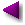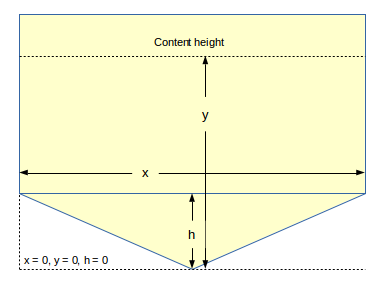Home | Mathematics | * Applied Mathematics | * Storage Tank Modeling | * TankCalc * TankFlow * TankProfiler Storage Container Mathematics TankProfiler Case History TankStepped TankStepped Java Source Listing Trapezoidal Storage Tanks Trapezoidal Storage Tanks: Java Listing User inquiries about TankCalc Volumes In DepthShare This Page
Common questions about tank volume computation

(double-click any word to see its definition)

If you have a question about TankCalc that might prove useful to others,
post it here and I will add it to this list.

Poor accuracy in table listing
I have used TankCalc for horizontal tank satisfactorily. But in vertical tank, calculated result shows same volume for different height/length. For me when I have entered Radius 94.7, Length 150 and r 11.5 all in cm. Then on computation [table generation], result for height 14 & 15 give same volume 63.34 [liters].

(The original post included a sample table with some random successive identical volume results for different height indices)
Okay, I see the problem. Please increase the number of cylinder integration steps to 400 (or more). The higher the number of integration steps, the more accurate the result. Go to the "Tune" tab and change the entry for "Cylinder integration steps" to a value substantially greater than 100.

In general, if you see table results of questionable accuracy or (as in this case) paired volume results in a table, changing the number of integration steps should be the first applied remedy.

The only reason for the default value of 100 is that this produces satisfactory accuracy in the majority of cases with reasonable program speed. Higher integration step entries improve accuracy but take more time to generate a table. If you have a fast computer, this issue may never come up for you, and you may want to use a larger default value.

The worst case involves the use of the slower inverse computation mode (height from volume) and a large integration steps entry. In such a case I might be offering the opposite advice — a smaller entry to save computation time.
Tank wider than tall
We are trying to calculate the volume in an Elliptical fuel vessel. The horizontal cylinder vessel Length is 2560mm. The vertical diameter is 1470mm, the Horizontal diameter is 2255mm, the Hemisphere caps left and right are, radius "r" is 175mm. Can this type of vessel be calculated on your tank calculator? Yes, sort of. Take the vertical dimension of the tank and perform all the usual computations. Then create a scaling factor (sf) created by dividing the horizontal by the vertical dimension:

sf = h/v (greater than one).

Then create a table of TankCalc results and multiply all the volume results by this ratio.

Worked example:
"The horizontal cylinder vessel Length is 2560mm. The vertical diameter is 1470mm, the Horizontal diameter is 2255mm, the Hemisphere caps left and right are, radius "r" is 175mm."

TankCalc entries for this tank (all millimeters):
• L = 2560
• R = 735 (diameter divided by 2)
• r = 175 (both left and right)
Full volume result: 4740.75 liters

Scaling Factor sf = 2255 / 1470 = 1.534

Adjusted full volume result: 7272.31 liters (4740.75 * 1.534)

Table procedure: Create a table of results in TankCalc, transfer the table to a spreadsheet, and multiply the volume results by the computed correction factor.
TankCalc versions 7.3 and higher have a scaling factor entry to accommodate this tank type. Use the above instructions, but enter the computed ratio into TankCalc itself.
80% Filling Rule

[ This exchange, which continues a conversation on other topics, refers to the liquid-phase storage safety rule that requires no more than 80% fill by volume. ]

Yes — with regard to the "80% filling rule.....", I would appreciate it if you could advise how to set this up. Okay. The first thing you need to know is that the equations I have written that give a volume for a sensor height have no reciprocal symbolic form — they can't be restated to provide a sensor height for a given volume. But that's exactly what we need. So we use a method called Root Finding internal to TankCalc. Root finding is a numerical and approximate method, but you can get any degree of numerical accuracy you require.

For this example, and just so we get the same numerical results, go to the TankCalc "Input" tab and select "Defaults" just under the tank picture. This resets all values to their defaults.

Now go to the "Compute" tab and click the "Height -> Volume" button at the upper left. The last result, at the bottom of the table, is for a completely filled tank, i.e. 100%, and for a sensor height of 72 inches that volume is 2115.07 gallons.

With a hand calculator, compute 80% of 2115.07 = 1692.06. Now, to get sensor heights for volumes, change modes by pressing the "Volume -> Height" button at the upper left. This mode uses the aforementioned root finder method to acquire sensor heights for volume arguments.

Now enter 1692.06 into the window that should be marked "Enter volume gallons" at the bottom of the display and press Enter. Directly adjacent to this entry will appear "53.20", which is the sensor height for 80% volume.

To test the accuracy of this result, reverse the process — press "Height -> Volume" again at the upper left to return to the default mode, and enter 53.20 into the single result window at the bottom of the display marked "Enter Height Inches" and press Enter. The result will be 1691.93.

Notice that this result isn't exactly the same as the original argument — this arises from the errors inherent in the numerical root finding method. I mention this now for two reasons — one, this error can be reduced, but two, that refinement has little practical significance, because it's already a small fraction of one percent:

1692.06 / 1691.93 = 1.000077

You can get a more accurate result by changing the "Cylinder integration steps", "End cap integration steps" and "Root finder epsilon" values on the "Input" tab, as well as the number of displayed decimal places, but there's really no point, because the default result is already much better than one can expect to get using field-acquired tank measurements and sensor readings.

The important thing about this exercise is not getting a particular result, but understanding why it works. Also it's important to know that most spreadsheets and programming languages can be configured to provide a root finder-derived result. So if you take the equations for tank volume from sensor height and put them into another environment, you should be able to get root-finder numerical results there also.
Boat Hull Cross-section
I would like to calculate the volume of a rectangular vessel with a two way slope on the floor, (not just from one side to the other) I would also like to calculate the volume from not just the extreme bottom but from any position on the slope.

Here's how I would proceed — for the sloped part, write an equation for the area of the sloped section as a triangle with the same angles (just use the height argument as the height of the triangle), then to get a volume for the computed area, simply multiply by the length of the sloped area.

Then, for the rectangular part, it's simply width times length times that section's height minus the sloped part. Then add that computed volume to the volume you already computed for the sloped part.

One more thing — a container with a two-way slope can be computed as though it has only one slope. Just figure out the width of the container at each vertical height. Once you've done that, it no longer matters how you picture it, because the widths at each height are the same whether there are one or two slopes. To see this, cut up some cards with different widths and stack them. You will see that it doesn't matter whether they're centered or the cards are lined up on one side, the volume is the same.

Just digesting your suggestions, what you say makes sense I will apply when I have some measurements for my vessel.

As it happens, after writing yesterday, I worked on this problem some more. My solution assumes a rectangular tank with a double-slope bottom section, sort of like the inside of a boat, but with an important difference — the Z axis must have the same size at all heights. Here's my solution:Figure 1

The tank has three dimensions — x, left-right on the page, y, up-down on the page, and z (not shown above), in and out from the plane of the page. The sloped shape at the bottom is limited to the x and y dimensions, the z dimension has the same size at all heights. And the y dimension's zero point is located at the bottom of the tank (bottom dashed line as shown above).

Incremental volumes for this tank are acquired in two separate solutions that depend on whether the y content height is greater than or equal to the "h" height value.

If the y content height is less than "h", the solution volume "v" is equal to:

\begin{equation} v = \frac{x y^2 z}{2h} \end{equation}

If the y content height is greater than or equal to "h", the solution volume has two parts:

Part one (the part of the tank above "h"):

\begin{equation} v = x (y-h) z \end{equation}

Part two (the part below "h", which is now constant):

\begin{equation} v = \frac{x h z}{2} \end{equation}

Simply add Part 1 to Part 2.

On that basis, the complete volume equation for y >= "h" is:

\begin{equation} v = [(y-h) x + \frac{x h}{2}] z \end{equation}

A typical embodiment for this solution would be a computer program that would first determine whether y is greater than or equal to "h", then select the correct method on that basis.

Here's an example Python program that solves for this tank shape — remember for this method to work, the Z dimension must have a fixed size:

Here is a plain-text listing for the above program.

 Home | Mathematics | * Applied Mathematics | * Storage Tank Modeling | * TankCalc * TankFlow * TankProfiler Storage Container Mathematics TankProfiler Case History TankStepped TankStepped Java Source Listing Trapezoidal Storage Tanks Trapezoidal Storage Tanks: Java Listing User inquiries about TankCalc Volumes In DepthShare This Page